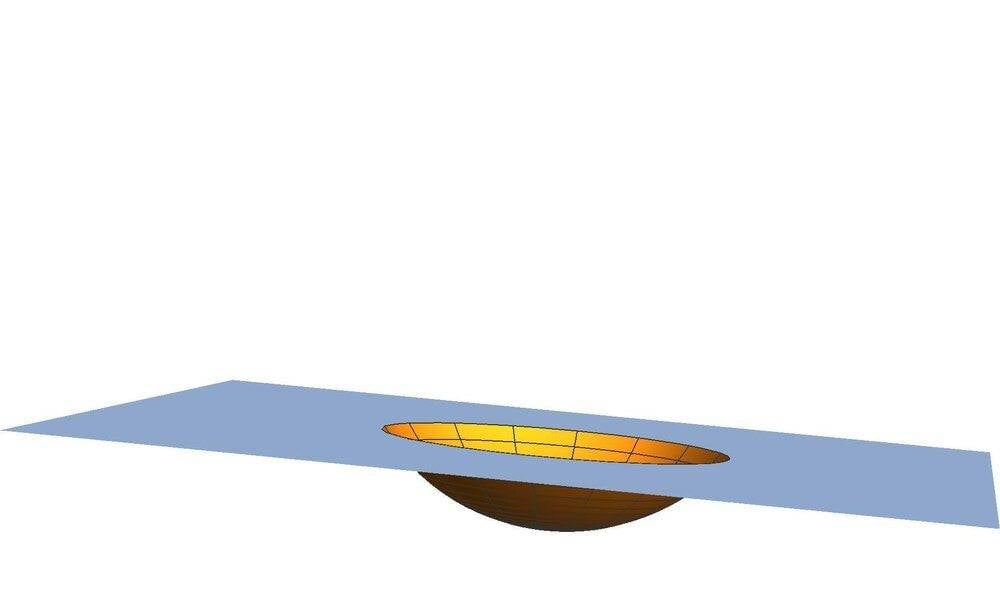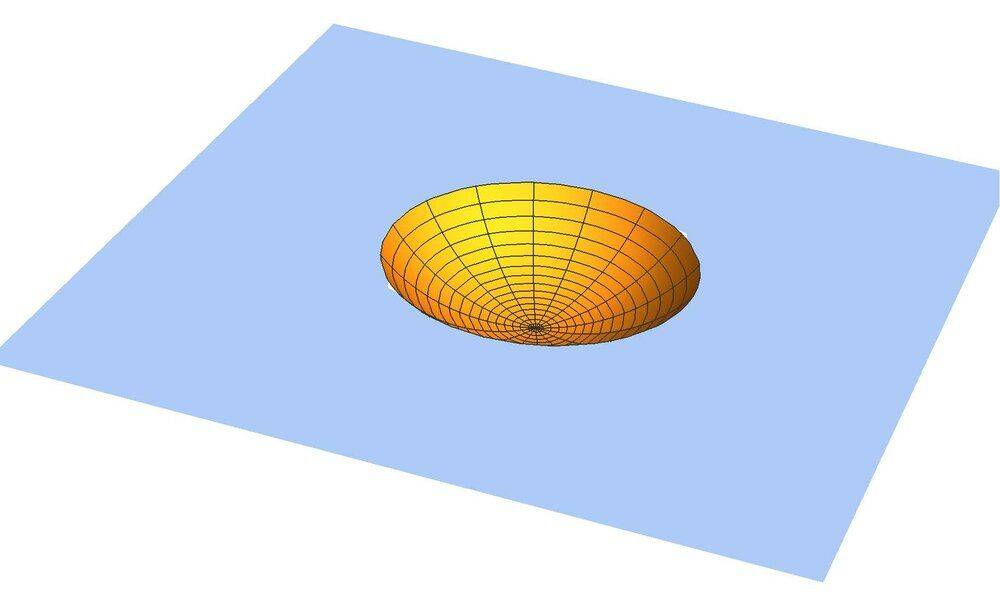# Heat conduction from an isotherm spherical cap

umby
On the surface of a semi-infinite solid, a point heat source releases a power ##q##; apart from this, the surface of the solid is adiabatic. The heat melts the solid so that a molten pool forms and grows. Let's hypothesize that the pool temperature is homogeneously equal to the melting temperature (hopefully this simplifies the problem) and the liquid and solid densities are the same. The melt pool exchanges heat with just the rest of the solid by conduction through its surface. A steady-state regime eventually is reached, in which q is equal to the heat flux through the surface of the pool. In this condition, what will the shape of the pool look like? Symmetry arguments suggest a spherical cap. What are its dimensions as function of q, density, thermal conductivity, melting temperature, and initial temperature of the solid? Is it possible to find the temperature field in the solid.

Homework Helper
Is this homework ?

I see a lot of inconcistencies in your scenario -- if I understand it correctly.
pool temperature is homogeneously equal to the melting temperature
meaning no heat transport takes place ?
A steady-state regime eventually is reached
And why would that be so ?

Mentor
Is this homework ?
Looks like @umby has been at PF for 5 years with nothing in the schoolwork forums so far. That would make me think it's not schoolwork, but it sure looks like it.

@umby if this is for your schoolwork, I can move it to the schoolwork forums for you. Either way you need to show us your work on the problem so far before we can be of help. Thanks.

umby
I apologize for the bad exposition of the problem. I will try to explain my thoughts with another example.
Let's suppose there is a heat point source of power ##q## in an infinite solid initially at temperature ##T_0##. This source melts part of the solid forming a spherical melt pool; the density does not change. If the temperature in the pool is homogeneous (high conduction rate, high conductive transport in the liquid) and does not exceed the melting temperature ##T_1##, I have an isotherm sphere of radius ##r_0## in an infinite medium at a given time. In this case, the temperature field outside the sphere is known to be:

##T=\frac{r_0 \left(T_1-T_0\right)}{r}+T_0##

The heat flux on the surface of the sphere is then:

##-k\frac{\partial T}{\partial r}\bigg| _{r=r_0}=k \frac{\left(T_1-T_0\right)}{r_0}##

The sphere will stop growing, namely the source will stop melting new solid, when ##q## will be equal to the heat flow through the surface. If we call ##r_{\max}## the sphere radius in this moment, then

##q=\frac{4 k \left(T_1-T_0\right) \pi r_{\max }^2}{r_{\max }}##

From which

##r_{\max }=\frac{q}{4 k\pi \left(T_1-T_0\right) }##

I call this steady-state because the spherical molten pool does not grow anymore and its radius remains equal to ##r_{max}##. In this case, it is probably easy to introduce a temperature gradient in the sphere as well and solve the problem, however the previous formula can provide a quick first estimate of the sphere radius in the proposed conditions. Is it correct to say that that that kind of stationary state is reached?

Mentor
Sure. It's the same problem, except that all the q goes to the cap instead of to each half.

Homework Helper
I apologize for the bad exposition of the problem. I will try to explain my thoughts with another example.
Let's suppose there is a heat point source of power ##q## in an infinite solid initially at temperature ##T_0##. This source melts part of the solid forming a spherical melt pool; the density does not change.
Problem statement is clear now, thanks. I don't think this example is any different from the original (symmetry)

If the temperature in the pool is homogeneous (high conduction rate, high conductive transport in the liquid) and does not exceed the melting temperature ##T_1##, I have an isotherm sphere of radius ##r_0## in an infinite medium at a given time.
No can do. As long as the heat source is on, heat will need to flow outward, so no isothermal sphere, no matter how high the conductivity.

There will be a radial temperature profile with a small temperature difference at the SL interface.

Bottom line is: what happens at the SL interface, where L side is by definition at ##T_L > T_m\ ## and S side at ##T_S <T_m\ ##. So far I ve been playing devil's advocate, but I can imagine q being dissipated outwards
without ##T_S## exceeding ##T_m##.
It's not a real steady state but the sphere stops growing.

Imagining is one thing; I will be convinced when I see it quantified

Happy to see Chet come in ! Any conclusive insight ?

##\ ##

umby
Problem statement is clear now, thanks. I don't think this example is any different from the original (symmetry)
In the case of the sphere, one independent variable: ##r##, but for the spherical cap both ##r## and ##\theta##.

umby
Bottom line is: what happens at the SL interface, where L side is by definition at and S side at . So far I ve been playing devil's advocate, but I can imagine q being dissipated outwards
without exceeding .
At the SL interface temperature is the melting temperature.

umby
It's not a real steady state but the sphere stops growing.
You have a steady-state if nothing changes with time.

Mentor
In the case of the sphere, one independent variable: ##r##, but for the spherical cap both ##r## and ##\theta##.
Not if the bottom is insulated. Then it is still only a function of r.

•umby
umby
Not if the bottom is insulated. Then it is still only a function of r.
Semi-infinite solid.

Mentor
Semi-infinite solid

I'm assuming that the semi-infinite solid is above my bottom plane.

•umby
umby
Returning to the spherical cap, in a spherical coordinate system centered at the "center" of the cap, the PDE is:
##\frac{\partial }{\partial r}\left(r^2\frac{\partial T}{\partial r}\right)+\frac{1}{\sin \theta }\frac{\partial }{\partial \theta }\left(\sin \theta \frac{\partial T}{\partial \theta }\right)=0##
With boundary conditions in dimensionless form given by:

##T=0##, ##r\to \infty##, this sets ##T## equal to the initial value far from the cap,

##T=1##, ##r=\frac{1}{2} \left(\sqrt{2} \sqrt{-p^2+(1-p)^2 \cos (2 \theta )+2 p+1}+2 (1-p) \cos (\theta )\right)##, this imposes ##T## on the cap

##\frac{\partial T}{\partial \theta}\bigg| _{\theta=\pi/2}=0##

##\frac{\partial T}{\partial \theta}\bigg| _{\theta=3\pi/2}=0##, those are the adiabatic conditions.
Do you think it is possible to find a solution to this problem?

Homework Helper
2022 Award
I got no LateX ...did you use ##? OK

Last edited:
Homework Helper
2022 Award
What happened to the spherical symmetry? Any plane thru the origin will be adiabatic automatically. Am I missing something?

•Chestermiller
Mentor
What happened to the spherical symmetry? Any plane thru the origin will be adiabatic automatically. Am I missing something?
That's what I've been trying to say.

•hutchphd
Homework Helper
At the SL interface temperature is the melting temperature.
Convice me ...

Mentor
Does this analysis take into account the heat of fusion of the solid?

Also, it is perfectly obvious to several of us that the solution is the same as for a point source in an infinite medium, meaning that the solution is not a function of ##\theta##.

umby
I try to upload an image of the surface of the semi-infinite solid. The spherical cap is isotherm while the rest of the surface is adiabatic. The semi-infinite solid is beneath the surface. I apologize for the misunderstandings.Last edited:
Homework Helper
2022 Award
Does this analysis take into account the heat of fusion of the solid?
I don't think that will matter steady state?

Yes, I can see that now. Thanks.

Last edited by a moderator:
•umby
umby
Is the cap meant to be a perfect hemisphere (it looks like less how much less?). To which post does this refer ?
I refer to the first post. The cap is not meant to be a hemisphere. In normalization, length dimensions were re-scaled by means of ##R## (the radius of the sphere from which the spherical cap is derived), this means that ##p##, the depth of the cap below the surface, can vary from 0 to 1 (0 = no cap, 1 = hemisphere). I would like to calculate the stationary temperature field in this semi-infinite solid, with this strange spherical cap at constant temperature ##T=T_1## on its surface (in dimensionless form ##T=1##). Far from the surface the temperature is ##T=T_0## (in dimensionless form ##T=0##). In a spherical coordinate system (physical convention, polar axis perpendicular to the surface) centered at the "center" of the cap, the dimensionless equations should be:
$$\frac{\partial }{\partial r}\left(r^2\frac{\partial T}{\partial r}\right)+\frac{1}{\sin \theta }\frac{\partial }{\partial \theta }\left(\sin \theta \frac{\partial T}{\partial \theta }\right)=0$$

B.C
##T=0##, ##r\to \infty## (##T## equal to the initial one far from the cap),
##T=1##, ##r=\frac{1}{2} \left(\sqrt{2} \sqrt{-p^2+(1-p)^2 \cos (2 \theta )+2 p+1}+2 (1-p) \cos (\theta )\right)## (##T## on the cap, this is the equation of the cap in the chosen reference system),
##\frac{\partial T}{\partial \theta}\bigg| _{\theta=\pi/2}=0## (adiabatic condition),
##\frac{\partial T}{\partial \theta}\bigg| _{\theta=\pi}=0## (symmetry conditions).

I hope that the problem formulation is correct. I have already tried to solve the problem hypothesizing ##T(r,\theta)=f(r) g(\theta)##, but this does not seem to give good results. I am sure on this point however.

Last edited:
•hutchphd